Next: Bibliography Up: Method of determination of Previous: Method of determination of

## Application of function Pearson7 for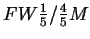method

Rozk3ad wielko¶ci ziaren z dopasowania Pearson7

Presentedmethod of GSD determination is only as precise as measurements of both widths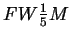and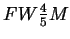. This precision can be raised by fitting an analytical curve to the experimental data being evaluated, then measurement of widths of the analytical (instead of experimental) curve. One of possible choices could be popular function Pearson7: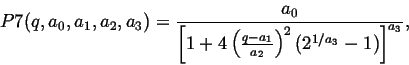(4)

where a0 is line intensity, a1 - line position, a2 i a3 are line widths. Putting a0=1 and a1=0 and comparing expression (4) to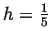and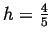we obtain equation for the width of Pearson7 curve at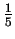and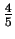of maximum:(5)

Interesting solutions of above equation are: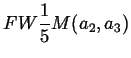=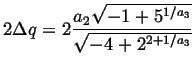(6)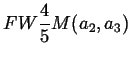=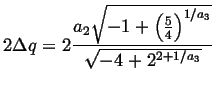(7)

Above expressions are functions of parameters a2 and a3, being immediate result of fitting in a crystallographic software (e.g. PeakFit). These values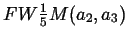and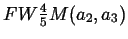can be placed in equations (3) and we obtain a recipe how to transform Pearson7 widths to the physical quantities of <R> and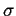, defining Grain Size Distribution: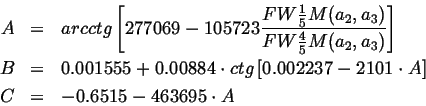=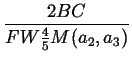(8)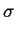=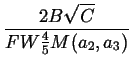Next: Bibliography Up: Method of determination of Previous: Method of determination of
roman pielaszek 2003-03-17